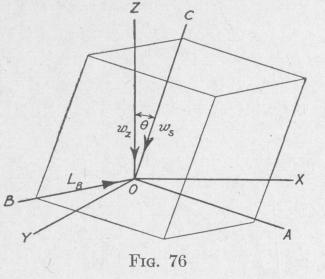Previous Page Index(For best results view this page as a PDF) Next Page

90   MOTION OF A SPINNING BODY

With the angular velocity of the movable reference frame ABCO, relative to the fixed frame of coordinate axes XYZO, as indicated in the diagrams, the rotation of the rigid frame ABCO produces changes in the magnitudes of the component angular momenta about the axes OA, OB, and OC. When the component angular momentum hB, Fig. 74, is turned with angular velocity wc, there is a change of angular momentum about the axis OA in the direction opposite hA. Thus, the rate of change of momentum hBWC, that is, the torque about the axis OA contributed by the angular velocity we is negative. Again, in Fig. 75, the turning of he with angular velocity WB produces angular momentum about the axis OA in the direction of hA, so that the torque hcwB is positive.

Proceeding in the same manner we find that the torque hAwc about the axis OB is positive whereas the torque hcwA about the same axis is negative. Similarly, the torque hBwA about the axis OC can be shown to be positive and the torque hAWB to be negative.

When the spin-velocity is constant and about the A-axis, and the precessional velocity is about the B-axis, and the resultant external torque is about the C-axis of a fixed system of coordinate axes OABC, then equations (70), (71), and (72) reduce to the expression for the Second Law of Gyrodynamics (55). This is evident if we substitute in (70), (71), and (72),

hA = hs, (constant), he = 0, and hB = KBWB, (constant), WB = wp and wA = we = 0

53. Components of the Torque Required to Maintain Constant Precession of a Body when the Precession-Axis is Inclined to the Spin-Axis. - Consider a symmetrical body spinning about an axis CO fixed with respect to a rigid frame of reference OABC, Fig. 76. The spin-axle CO with the attached rigid reference frame rotates with angular velocity w, about an axis ZO of a fixed system of rectangular coordinates OX YZ. The spin-axle is at a constant inclination 0 to the axis ZO. At the instant considered, let AO be in the plane ZOC. Then BO is perpendicular to ZO. The

required values of the components with respect to the axes A0, BO, and CO, respectively, of the torque required to maintain a constant velocity of precession of a symmetrical body spinning at constant angular speed w, about an axis inclined at a constant angle 0 to the axis of precession, are obtained from (70), (71), and (72) by substitution of the proper values now to be determined.

c:_...- irn ,.t 4L   m c .   4!-   +.+o. ,n;+h --j

MOTION OF A SPINNING BODY   91

velocity w, about ZO of the fixed system of coordinates, the components of wz about AO, BO, and CO are, respectively:

WA = -wz sin O, WB = 0, and we = w, cos 0   (73)

Now we shall determine the components of the angular momentum of the spinning body relative to the axes AO, BO, .and

CO. The component angular

velocity of the spinning body about any line equals the sum of the components of w, and w, about that line. The component angular velocities of the spinning body relative to the rectangular axes AO, BO, and CO, are (0 - wz sin 0), (0 + 0), and (w, + wZ cos 0), respectively. Representing the moments of inertia of the

spinning body relative to the axes AO, BO, and CO by KA, KB, and Kc, respectively, the values of the angular momenta of the spinning body about these axes are seen to be

hA = - KAw, sin 0, hB = 0, and he = Kc(w, + wZ cos 0)

(74)

Assuming that the velocities of spin and precession are constant and the position of the spin-axle relative to the axes AO, BO, and CO, is constant, it follows that

dhA _ 0' dhB = 0 and dhc = 0   (75)

d d   dt

Substituting in (70), (71), and (72) the values given in (73), (74 and (75), we find that the torques due to the external forces actii upon the spinning body about the axes AO, BO, and CO are, r spectively,

LA = 0

LB = - KAw'2 sin 0 cos 0 + Kc(w, + wz cos 0)w, sin 0 (7

Lc = 0

Consequently, for steady spin and steady precession about an a3l inclined to the axis of spin, there must be zero torque about al line in the plane of the spin and precession axes. but there muPrevious Page Index(For best results view this page as a PDF) Next Page